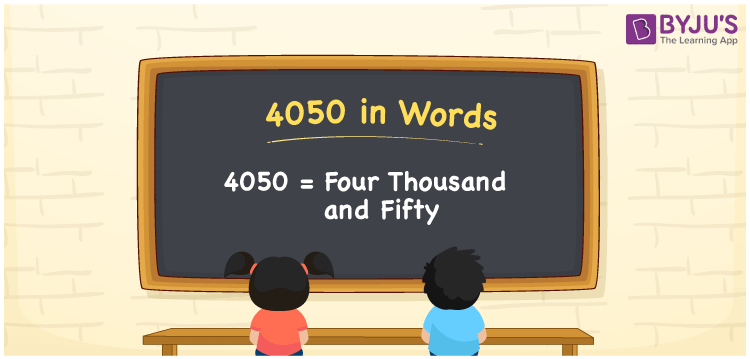# 4050 in Words

The number 4050 is written as Four Thousand and Fifty. We can write the number names using ones, tens, hundreds, thousands places of the number. Hence, a place value table is helpful while writing the numbers in words. For example, If you purchased two cotton sarees worth Rs. 4050, you can say, “I purchased two cotton sarees worth Rupees Four Thousand and Fifty”. We know that 4050 is a cardinal number since it shows an exact value.

 4050 in Words Four Thousand and Fifty Four Thousand and Fifty in numerical form 4050

## 4050 in English Words

Using the English alphabet, we can write the numbers in words. Thus, 4050 in English is written as Four Thousand and Fifty.## How to Write 4050 in Words?

To write the number 4050 in English words, first, determine the place value of each digit in the number. The place value chart for the number 4050 is shown below:

 Thousands Hundreds Tens Ones 4 0 5 0

Hence, we can write the expanded form as:

4 x Thousand + 0 x Hundred + 5 x Ten + 0 x One

= 4 x 1000 + 0 x 100 + 5 x 10 + 0 x 1

= 4000 + 0 + 50 + 0

= 4000 + 50

= 4050

= Four Thousand and Fifty

Thus, 4050 in words is written as Four Thousand and Fifty

Interesting way of writing 4050 in words

4 = Four

40 = Forty

405 = Four Hundred and Five

4050 = Four Thousand and Fifty

Hence, the word form of the number 4050 is Four Thousand and Fifty.

4050 is a natural number that is one greater than 4049 and one less than 4051

• 4050 in words – Four Thousand and Fifty
• Is 4050 an odd number? – No
• Is 4050 an even number? – Yes
• Is 4050 a perfect square number? – No
• Is 4050 a perfect cube number? – No
• Is 4050 a prime number? – No
• Is 4050 a composite number? – Yes

## Frequently Asked Questions on 4050 in Words

Q1

### How do you write 4050 in words?

4050 in words is written as Four Thousand and Fifty.
Q2

### Simplify 3000 + 1050, and express in words.

Simplifying 3000 + 1050, we get 4050. Therefore, 4050 in words is Four Thousand and Fifty.
Q3

### Write Four Thousand and Fifty in numbers.

Four Thousand and Fifty in numbers is 4050.